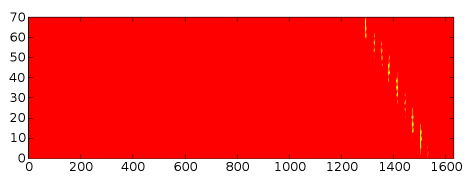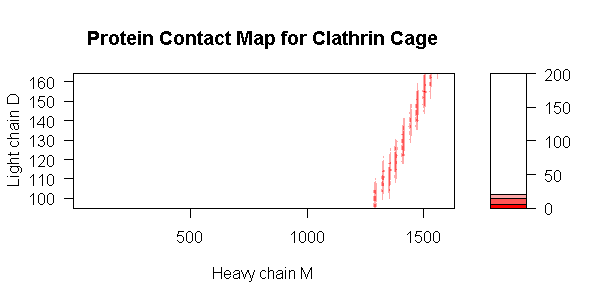# Protein Contact Maps using Biopython

When working with protein 3D structures, a contact map is usually defined as a binary matrix with the rows and columns representing the residues of two different chains. Each matrix element is one/True if the alpha carbon distance between the associated residues is less than some threshold (such as 6 angstroms), or zero/False if the residues are far away.

This means that the first step in calculating a contact map between to protein chains, is to calculate the alpha carbon distance between pair of their residues. BioPython's Bio.PDB module includes code to load PDB files and calculate these distances.

## An example PDB fileI have choosen to look at PDB file 1XI4, which is the clathrin cage lattice (described in more detail as the PDB's April 2007 Molecule of the Month). If you download the default PDB file (just over 1MB) you only get part of the complex ball structure made up of just nine clathrin heavy chains (Chains A to I in the PDB file) and nine clathrin light chains (Chains J to R). For drawing pretty pictures you want to download and uncompress the Biological Unit Coordinates instead (once uncompressed this is a 14 MB file). In both cases, only the alpha carbon atoms are listed in the PDB file, and there are no annotated alpha helices or beta sheets. This means that most of the fancy protein visualisations available in RasMol or VMD won't work - so the illustration on the left is from the PDB website.

## Calculating the distances and contact map

As with most python scripts, this one starts by importing some libraries, and setting up some constants:

```import Bio.PDB
import numpy

pdb_code = "1XI4"
pdb_filename = "1XI4.pdb" #not the full cage!```

Now we define a simple function which returns the distance between two residues' alpha carbon atoms, and a second function which uses this to calculate an entire distance matrix:

```def calc_residue_dist(residue_one, residue_two) :
"""Returns the C-alpha distance between two residues"""
diff_vector  = residue_one["CA"].coord - residue_two["CA"].coord
return numpy.sqrt(numpy.sum(diff_vector * diff_vector))

def calc_dist_matrix(chain_one, chain_two) :
"""Returns a matrix of C-alpha distances between two chains"""
for row, residue_one in enumerate(chain_one) :
for col, residue_two in enumerate(chain_two) :

Then the PDB file is read into the variable structure using Bio.PDB.PDBParser(). This provides a list of the models in the PDB file (in this case, only one as NMR was not used).

```structure = Bio.PDB.PDBParser().get_structure(pdb_code, pdb_filename)
model = structure```

Within this model there are in fact 18 chains (9 heavy and 9 light). The following code calculates the distance matrix between light chain D and heavy chain M - chosen because they are in close contact (and also positioned in the centre of the sub-unit of the full cage represented in the PDB file).

```dist_matrix = calc_dist_matrix(model["D"], model["M"])
contact_map = dist_matrix < 12.0```

The final line turned the distance matrix (held as a float array) into contact map (as a logical array) using a threshold of 12 angstroms.

Now, one obvious question is what is the range of distances in our matrix? You can try using the python built in min() and max() functions but the don't give the expected behaviour for Numeric arrays. Instead, we must use numpy's own functions:

```print "Minimum distance", numpy.min(dist_matrix)
print "Maximum distance", numpy.max(dist_matrix)
```

This gives the following output:

```Minimum distance 4.62857341766
Maximum distance 201.617782593```

The closest residues turn out to be residue Asn155 in light chain D, and Leu1504 in heavy chain M. In fact there are only a couple of pairs closer than six angstroms - but its not very easy to see this from a massive matrix like this.

## Drawing the distance matrix in Python

I'll show another method in a moment, but first lets use the python library Matplotlib to do some plotting:

```import pylab
pylab.matshow(numpy.transpose(dist_matrix))
pylab.colorbar()
pylab.show()```As you can see, the light chain (vertical axis) is in contact with only the C-terminal region of the heavy chain (horizontal axis). You can also see some banding in the distance plot, which you can probably explain in terms of the secondary structure.

You might want to look at pylab.pcolor() instead of pylab.matshow(), for one thing the y-axis runs the other way up.

Now lets show the contact map (which is a boolean matrix, so with only two values a colour key is a bit redundant), and while we're at it, lets change the colour scheme:

```pylab.autumn()
pylab.imshow(numpy.transpose(contact_map))
pylab.show()```Pretty! However, the vertical axis needs relabelling for some reason...

## Drawing a contour plot of the distances

This is really overkill on this example where plotting an array of little coloured squares is more than enough, but I went on to show the distance map as a contour plot. It seemed like a logical next step - especially as I have already worked out how to do draw this sort of thing in R (e.g. Contour Plots of Matrix Data in R) and you can call R from python using rpy.

This bit of code was used to turn the distance matrix into a contour plot:

This used to work fine with Numeric instead of numpy, but perhaps my copy of rpy is out of date as it complains "no proper 'z' matrix specified".

```import rpy
rpy.r.library("gplots")
rpy.r.png("1XI4_D-M.png", width=600, height=300)
rpy.r.filled_contour(x=rpy.r("1:1630"), \
# residue numbers in heavy chain
y=rpy.r("95:164"), \
# residue numbers in light chain
z=dist_matrix, \
xlab="Heavy chain M", \
ylab="Light chain D", \
levels=[0,6,14,20,200], \
col=rpy.r.colorpanel(4, "red", "white"),
main="Protein Contact Map for Clathrin Cage")
rpy.r.dev_off() # close the image file```

And here is the resulting image, notice again the all of the light chain is in contact with the C-terminal region of the heavy chain - maybe it would be more interesting to produce a plot of just that region... The colour key changes at 6, 14, and 20 angstroms: